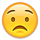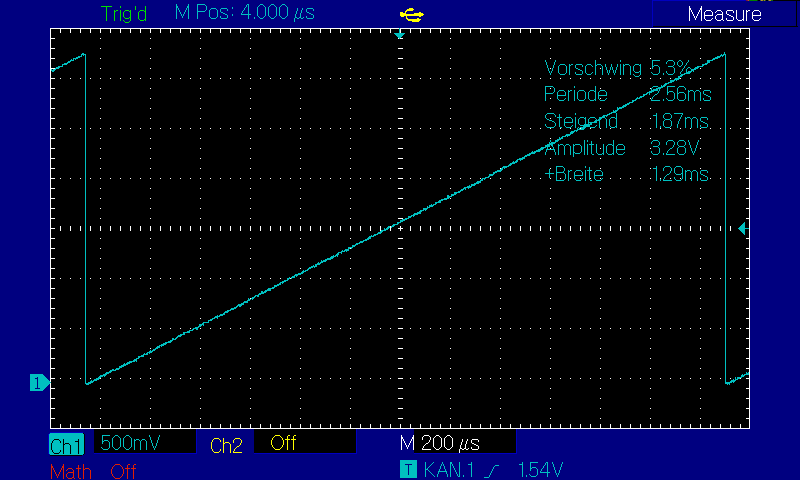P2 PASM SIN 16Bit - Page 2 — Parallax Forums

# P2 PASM SIN 16Bit

## Comments

• You definitely want to read the hardware doc. Come back to the instruction sheet later.

• For the base instructions, the Propeller 1 manual explains every instruction in great detail. There were documents done while P2 was being designed but they changed so often this info became lost/broken.

• I have learned to appreciate the P1 manual as a great help. Most of my projects are in PASM. The other programming languages hardly played a role.

It's a pity that the basics of the propeller are so poorly maintained. Perhaps someone will take pity on me.

• edited 2021-03-18 00:03

First test "create a sawtooth" completed.
Some values are not as described DD & MMMMM both values should always be 0 at PPP = 101.
But it could also be that Google translates something wrong.```CON
_clkfreq        =   256_000_000   '                                                                    '
'                                                                                                      '
pin1            =   0             '                                                                    '
'                                                                                                      '
DAT     org                       '                                                                    '
asmclk                    '                                                                    '
'------------------------------------------------------------------------------------------------------'
' DAC                                                                                                  '
'------------------------------------------------------------------------------------------------------'
dac1                              '                                                                    '
wrpin   dac,#pin1         '                                                                    '
wxpin   #8,#pin1          '                                                                    '
dirh    #pin1 addpins 1   'SetDAC                                                              '
loop1                             '                                                                    '
wypin   outw,#pin1        '                                                                    '
waitse1                   'wait for new period
add     outw, #\$1                                                '
jmp     #loop1                       '                                                                    '
'                                 '                                                                    '
'------------------------------------------------------------------------------------------------------'
' Variabeln                                                                                            '
'------------------------------------------------------------------------------------------------------'
outw    long  0
'------------------------------------------------------------------------------------------------------'
'  Das Y-Register ist ein 32-Bit-Smartpin-Parameter. Einige Smartpin-Modi verwenden nicht alle 32 Bits.'
'  In diesem Fall sind es nur 16-Bit ohne Vorzeichen. Die oberen 8 Bits von Y werden direkt für den    '
'  DAC-Ausgang verwendet. Die unteren 8 Bits werden mit einem 8-Bit-Timer/RND verglichen, um den       '
'  erweiterten Dither zu erzeugen.                                                                     '
'------------------------------------------------------------------------------------------------------'
' DAC config                                                                                           '
'------------------------------------------------------------------------------------------------------'
'            bit 30    25     20     15   10      5     0                                              '
'                 |     |      |      |    |      |     |                                              '
'         D/# = %AAAA_BBBB_FFF_PPP_VV_DDDDDDDD_TT_MMMMM_0                                              '
dac     long    %0000_0000_000_101_00_00000000_01_00010_0   '                                          '
' AAAA        = %0000                                                                                  '
' BBBB        = %0000                                                                                  '
' FFF         = %000                                                                                   '
' PPP         = %101    = DAC_MODE (%TT = 00 and %MMMMM = 00000), 8-bit flash, registered              '
'                         OUT enables PinA ADC (ADC config %011), sysclocked bitstream on IN           '
'                         DIR enables PinA DAC output                                                  '
' VV          = 00      = PinA DAC config  990 ohm, 3.3 volt range                                     '
' DDDDDDDD    =         = DAC level                                                                    '
' TT          = %00     = --> PPP                                                                      '
' MMMMM       = %00000  = --> PPP                                                                      '
'                                                                                                      '
'----------------------------------------------------------------------------------------------------- '

```• edited 2021-03-18 06:02

@pic18f2550 said:
First test "create a sawtooth" completed.
Some values are not as described DD & MMMMM both values should always be 0 at PPP = 101.
But it could also be that Google translates something wrong.I can imagine the translation would be fraught for even an experienced human. Electronics and processors and computing have many borrowed and overused and even misused terms to describe functions.

As for D and M. It's an either-or case. There's actually four distinct ways to control each pin's DAC level.

• Either you can set it directly in the WRPIN instruction by leaving T=0 and M=0 and by filling D with the desired level. This mode isn't named specifically, it's common with DAC_MODE.
• Or you can set T=1 and M=0 and fill D with the cog number that will set the DAC level. This is called COG_DAC mode. This is needed for audio/video streamer output. The SETDACS instruction uses this too.
• Or you can set T=2 and M=0 and fill D with dual 4-bit levels. This is BIT_DAC mode. T=3 is a variant of this.
• Or finally, you can set T=1 and M from 1 to 3. D is not used. This is SMART_DAC mode, and is the one you've just used. This allows the smartpin to control the DAC level.

PS: There is a sort of fifth way, where a non-DAC smartpin mode can toggle the BIT_DAC levels. Set M above 3 and P=%101.... It combines the third and fourth ways together. It's unnamed but SMART_BITDAC would suit. EDIT: Ha, this way uses both M and D together.• PPS: Here's a link for a schematic I guessed at for the routes to one DAC. In particular it shows how BIT_DAC mode always needs the two levels set in D - https://forums.parallax.com/discussion/comment/1495181/#Comment_1495181

• I have a few questions about qrotate.

```qrotate v,t
getqy x
```

Does this correspond to x = v * sin( t ) ?

What is the range of values of (t) for a sine full wave = λ
In what order of magnitude (bit width and format ) are the individual sine values held before scaling (v)?
(For which v value is no calculation needed internally).

• @pic18f2550 said:
I have a few questions about qrotate.

```qrotate v,t
getqy x
```

Does this correspond to x = v * sin( t ) ?

What is the range of values of (t) for a sine full wave = λ
In what order of magnitude (bit width and format ) are the individual sine values held before scaling (v)?
(For which v value is no calculation needed internally).

Yes, should be. When sin(t) = 1, x is exactly equal to v. You could think of it as any fixed-point format, it really doesn't matter. The calculation happens at full 32 bit precision.

• edited 2021-03-23 12:09

Ok "v" and "x" are thus clarified.
The value range of "t" is still open.

This is important because I want to generate phase-shifted signals.

There is nothing useful about it in the documentation.
"Begin CORDIC rotation of point (D, SETQ value or 32'b0) by angle S. GETQX/GETQY retrieves X/Y."

Y = SIN(2 * 3,14...) = 0
Y = SIN(360°) = 0• edited 2021-03-23 14:21

Docs say:

unsigned 32-bit angle, where \$00000000..\$FFFFFFFF = 0..359.9999999 degrees

So t = \$4000_0000 (90 deg) should give you v == x.

EDIT: Doh! Corrected to 90 deg.• (OO)
Hats off, I didn't expect that.

• edited 2021-03-24 21:50

Now the formula of pic33 also makes sense.

```f1 long round(freql * 65536.0 * 65536.0 * 256.0 / float(clkfreq_))

```

freql is the frequency to generate

65536.0 * 65536.0 stands for the sine full wave

256.0 are the sample clocks of the DAC.

Since only the value of the sine wave is greater than 32 bits, I can't get there with a simple calculation in PASM.

I need a shortcut.

```The frequency is excluded first so I can generate a constant.

K = \$FFFF * \$FFFF * \$FF / 256_000_000
= \$FFFFFFFF / 256_000_000
= 4_294

Cross check :
4294 * 256_000_000 = \$FFF13D8000

```

Full of the face.

I need a better calculator.• With _clkfreq = 268_437_500 everything looks better.

The value comes closer to the multiple of 2.

• edited 2021-03-24 11:47

The Cordic's multiply produces a 64-bit result and its divide takes a 64-bit dividend. You'll note Chip provided a convenient built in Spin2 function called muldiv64() that gives an accurate 32-bit integer result of a concatenated multiply then divide of any three 32-bit values. It is making use of this Cordic capability. The Cordic is a nice piece of hardware.

• However, the divident is already 43 bits large.
If I have read this correctly, it may only be 32 bits.

• The divider is 32-bit. The dividend can be 64-bit.

• K1 := 4 * \$FFFF * \$FFFF * \$FF / 268_437_500

K1 := muldiv64( \$3_FF , \$FF_FFFF_FF , 268_437_500 )

Is that right?

• edited 2021-03-24 22:15

Yeah, something like that. Actually, given what this case is doing, you can probably cheat and just use the QFRAC cordic command since it pre-stacks the bottom 32 bits of the dividend. `QFRAC #256,clkfreq`

• Hello,
what happens if I consume more computation time (code) than the period has.

```dat     org
asmclk
wrpin   dac,#left
wxpin   #256,#left
dirh    #left addpins 1
setse1  #%001<<6 + left

loop    add     p1,f1           'calculate right sample
qrotate amp,p1
getqx   x
bitnot  x,#15
wypin   x,#left
........... 200 x Code .........
waitse1                 'wait for new period
jmp     #loop          'loop

```
1. waitse1 waits forever and ever.

2. waitse1 syncronizes normally with the 2nd period.

• edited 2021-03-25 15:11

If the event has already before the WAIT instruction, it doesn't wait at all.

If you want it to wait for the next period, do a POLLSE1 (to clear the event flag), then an AKPIN (to clear the smartpin IN) and then the WAITSE1.
If you want it to hang, just do a POLLSE1 before the WAITSE1.

• Okay, I'll have to come up with something else.
I can not say exactly how long it takes due to the nesting of the code. (self modifying code)

I will probably connect two pins to be able to syncronize the starts.

• A set event flag is cleared only when you execute certain instructions, eg: WAITSE1 clears the SE1 event flag.

Irrespective of the WAITxxx behaviour, the smartpin is going to skip a sample - retaining existing level. The WYPIN will be buffered for whatever sample period is next. The opposite effect of executing WYPIN too often.

• edited 2021-03-26 09:31

Two things that caught my eye:

1. "getqx x" does not return the sine value but the cosine value.
Correctly it must be called "getqy x".

2. the formula "f1 long round(freql * 65536.0 * 65536.0 * 256.0 / float(clkfreq_))" must be wrong.
I create a frequency of 2,9kHz instead of the desired 1khz.

About point 2 I have to think a bit more.• edited 2021-03-26 11:27

This is how it should actually work.
I'll test it this evening.

```DAC_Sample := clkfreq_ / 256
F1 := Frequency * ( \$1_0000_0000 / DAC_Sample )
```
• edited 2021-03-26 11:43
```MOV pa, freql
SHL pa, #8     'multiply by 256
QFRAC pa, clkfreq
GETQX f1
```
• Doesn't that cause an overflow? (32bit)

```freql = 268,437,500 = \$1000_07FC
freql * 256 = 68,720,000,000 = \$10_0007_FC00
```

What is "pa" for a register?

• That looks like the sysclock frequency, clkfreq. What are you wanting for freql?

• edited 2021-03-26 12:57

PA is another predefined label for a cog general register, like PTRA. I use PA and PB as scratch registers.

• Sorry, freql is a value from an array.

```clkfreq = 268.437.500 Hz = \$1000_07FC               Here it would still fit.
clkfreq * 256 = 68.720.000.000 Hz = \$10_0007_FC00   Hier aber nicht.
^^             Overflow
```
Sign In or Register to comment.